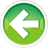# 8.14 — Struct passing and miscellany

Consider an employee represented by 3 loose variables:

If we want to pass this employee to a function, we have to pass three variables:

While passing 3 variables isn’t that bad, consider a struct with 10 or 12 members. Passing each variable independently would be time consuming and error prone. Additionally, if we ever add a new attribute to our employee (e.g. name), we now have to modify all the functions and function calls to accept the new parameter & argument!

Fortunately, we can pass entire structs to functions.

Passing structs (by reference)

A big advantage of using structs over individual variables is that we can pass the entire struct to a function that needs to work with the members:

In the above example, we pass an entire Employee struct to printInformation() (twice, once for `joe` and once for `frank`). Note that because structs are class types, we generally pass them by const reference to avoid making an unnecessary copy!

Furthermore, if we ever decide to add new members to our Employee struct, we will not have to change the function declaration or function call!

The above program outputs:

```ID:   14
Age:  32
Wage: 24.15

ID:   15
Age:  28
Wage: 18.27
```

Returning structs

Consider the case where we have a function that needs to return a point in 3-dimensional Cartesian space. Such a point has 3 attributes: an x coordinate, a y coordinate, and a z coordinate. But functions can only return one value. So how do we return all 3 coordinates back the user?

One common way is to return a struct.

This prints:

```The point is zero
```

Note that we’ll generally return structs by value, so as not to return a dangling reference.

Returning temporary structs

In the `getZeroPoint` function above, we create a new named object (`temp`) to do nothing but return it. We can improve this by returning a temporary (unnamed) object instead:

Note how much cleaner this is (one line vs two, and no need to understand whether `temp` is used more than once).

In the case where the function returns an explicit type (e.g. `Point3d`) rather than `auto`, we can omit the type in the return statement:

Also note that since in this case we’re returning all zero values, we can use empty braces to zero-initialize all the member:

Nested structs

Structs can contain other structs. For example:

In this case, if we wanted to know what the CEO’s salary was, we simply use the member selection operator twice: `myCompany.CEO.wage;`

This selects the `CEO` member from `myCompany`, and then selects the `wage` member from within `CEO`.

Note that our list-initialization contains a nested list for the nested struct.

Struct size and data structure alignment

Typically, the size of a struct is the sum of the size of all its members, but not always!

Consider the Employee struct. On many platforms, a short is 2 bytes, an int is 4 bytes, and a double is 8 bytes, so we’d expect Employee to be 2 + 4 + 8 = 14 bytes. To find out the exact size of Employee, we can use the sizeof operator:

On the author’s machine, this prints:

```The size of Employee is 16
```

It turns out, we can only say that the size of a struct will be at least as large as the size of all the variables it contains. But it could be larger! For performance reasons, the compiler will sometimes add gaps into structures (this is called padding).

In the Employee struct above, the compiler is invisibly adding 2 bytes of padding after member id, making the size of the structure 16 bytes instead of 14.

Accessing structs across multiple files

As a program-defined type, any file that wants to use a struct type needs to be able to access the definition of the struct. For this reason, struct definitions are typically put in header files, and #included anywhere they are needed.

Struct variables behave like normal variables, so they can be given external linkage and accessed across multiple files just like any normal variable can.

Structs are an important building block

Structs are very important in C++, as understanding structs is the first major step towards object-oriented programming! Later on in these tutorials, you’ll learn about another aggregate data type called a class, which is built on top of structs. Understanding structs well will help make the transition to classes that much easier.

Quiz time

Question #1

You are running a website, and you are trying to keep track of how much money you make per day from advertising. Declare an advertising struct that keeps track of how many ads you’ve shown to readers, what percentage of ads were clicked on by users, and how much you earned on average from each ad that was clicked. Read in values for each of these fields from the user. Pass the advertising struct to a function that prints each of the values, and then calculates how much you made for that day (multiply all 3 fields together).

Show Solution

Question #2

Create a struct to hold a fraction. The struct should have an integer numerator and an integer denominator member. Declare 2 fraction variables and read them in from the user. Write a function called multiply that takes both fractions, multiplies them together, and prints the result out as a decimal number. You do not need to reduce the fraction to its lowest terms.

Show Solution7.11 -- Introduction to class typesIndex8.13 -- Struct initialization